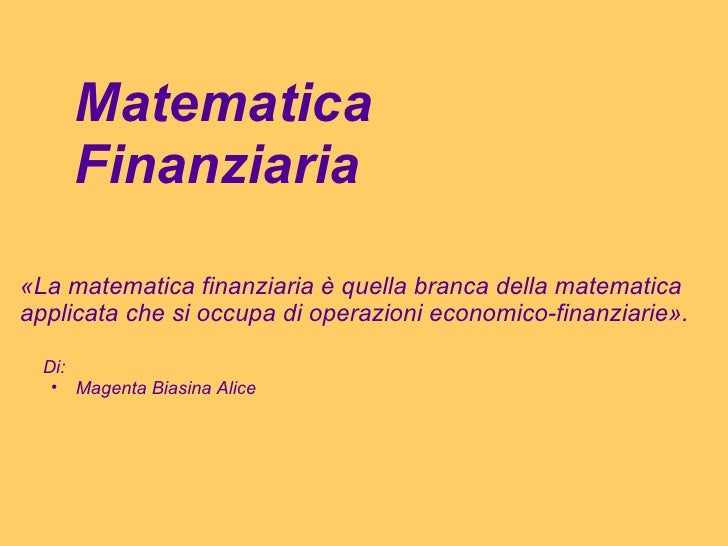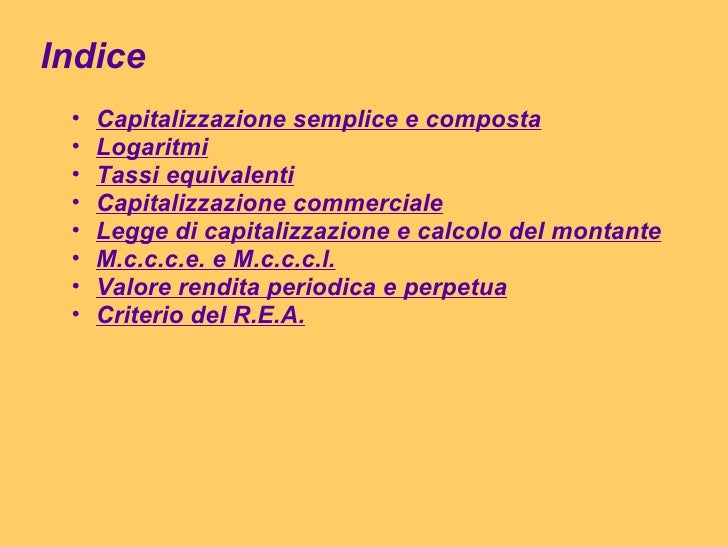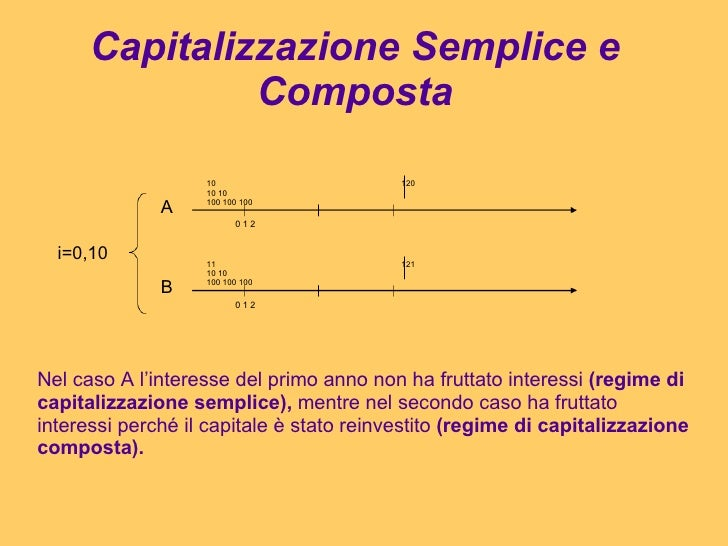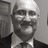Successfully reported this slideshow.Upcoming SlideShare
×

ofUpcoming SlideShare
01statistica medie
Next

Share

# Matematica finanziaria

a cura di Alice Magenta Biasina

See all

See all

### Matematica finanziaria

1. 1. Matematica Finanziaria «La matematica finanziaria è quella branca della matematica applicata che si occupa di operazioni economico-finanziarie». <ul><li>Di: </li></ul><ul><ul><li>Magenta Biasina Alice </li></ul></ul>
2. 2. Indice <ul><ul><li>Capitalizzazione semplice e composta </li></ul></ul><ul><ul><li>Logaritmi </li></ul></ul><ul><ul><li>Tassi equivalenti </li></ul></ul><ul><ul><li>Capitalizzazione commerciale </li></ul></ul><ul><ul><li>Legge di capitalizzazione e calcolo del montante </li></ul></ul><ul><ul><li>M.c.c.c.e. e M.c.c.c.l. </li></ul></ul><ul><ul><li>Valore rendita periodica e perpetua </li></ul></ul><ul><ul><li>Criterio del R.E.A. </li></ul></ul>
3. 3. Capitalizzazione Semplice e Composta 10 10 10 100 100 100 120 11 10 10 100 100 100 121 0 1 2 0 1 2 A B i=0,10 Nel caso A l’interesse del primo anno non ha fruttato interessi (regime di capitalizzazione semplice), mentre nel secondo caso ha fruttato interessi perché il capitale è stato reinvestito (regime di capitalizzazione composta).
4. 4. <ul><li>Capitalizzazione semplice </li></ul>M=C(1+i∙t) Fattore di capitalizzazione semplice: serve per portare avanti un capitale C nel tempo C=M(1/(1+i∙t)) Fattore di capitalizzazione di sconto semplice o razionale C M 1+i ∙ t 1/1∙i ∙t <ul><ul><li>Capitalizzazione composta </li></ul></ul>M=C(1+i)t C=M(1+i)-t Fattore di capitalizzazione composta C M (1+i)t (1+i) -t
5. 5. <ul><li>Grafico dei due montanti </li></ul>M =(1+i)t M =(1∙i ∙t) x y 0 < t < 1 c. s. t = 1 c.s. = c.c. t > 1 c.c.
6. 6. Logaritmi Il logaritmo di un numero “N” maggiore di zero in una base “a” positiva e diversa da 1 è l’esponente “x” a cui dobbiamo elevare la base “a” per ottenere “N”. LogaN=x ax= N N > o a > o a ≠ 1 <ul><ul><li>Proprietà dei logaritmi </li></ul></ul><ul><ul><li>Logabc = logab + logac </li></ul></ul><ul><ul><li>Logab/c = logab – logac </li></ul></ul><ul><ul><li>Logabc = c logab </li></ul></ul><ul><ul><li>Logab = logcb / logca </li></ul></ul>Es: Log28= 3
7. 7. <ul><ul><li>Grafici di funzioni logaritmiche </li></ul></ul><ul><ul><li>y = logax a > 1 </li></ul></ul><ul><li>x -> 0t y -> -∞ </li></ul><ul><li>x -> +∞ y -> +∞ </li></ul>0 y x 1 2) y = logax 0 < a < 1 x -> 0t y -> +∞ x -> +∞ y -> -∞ 1 0 y x
8. 8. 3) y = a x a > 1 x -> -∞ y -> 0 x -> +∞ y -> +∞ <ul><ul><li>Grafici di funzioni esponenziali </li></ul></ul>0 y x 1 4) y = a x 0 < a < 1 x -> +∞ y -> 0 x -> -∞ y -> +∞ 0 y x 1
9. 9. Tassi Equivalenti i= tasso annuo i4= tasso trimestrale i2= tasso semestrale i6= tasso bimestrale i3= tasso quadrimestrale i12= tasso mensile <ul><ul><li>Anno -> Periodo i=0,08 i3? </li></ul></ul><ul><li>(1+i3)3=1,08 i3= 3√1,08 -1=0,026 i3=2,6% </li></ul><ul><ul><li>Periodo -> Anno i4=0,05 i? </li></ul></ul><ul><li>1+i=(1,05)4 i=1,054-1 i=21,55% </li></ul><ul><ul><li>Periodo1 -> Periodo2 i4=0,05 i6? </li></ul></ul><ul><li>(1+i6)3= 1,052 i6=3√1,052 -1 i6=3,31% </li></ul>
10. 10. Capitalizzazione Commerciale S= M∙d∙t C= M(1-d∙t) M= C(1/(1-d∙t)) d= tasso di sconto commerciale C M 1/(1-d ∙ t) 1-d∙t Es: M= 1000 C= 1000(1-0,012(2+3/12)) d= 0,012 C= 973 € t= 2 anni e 3 mesi C= ?
11. 11. Legge di capitalizzazione e calcolo del montante f(t)= 1+i∙t 1) f(0)= 1 oppure f(0)= c 2) f(t) crescente i= coefficiente angolare f(0)= 1+0 i > 0 f(t) crescente LEGGE DI CAPITALIZZAZIONE i= 0,04 t=3 f(3)= 1+0,04∙3= 1,12 montante Es: f(t)= (1+i)t f(0)= (1+i)0 = 1 f(t)= crescente in quanto funzione del tipo ax con a>1 i= 0,05 t= 4 f(4)= 1,054= 1,2155
12. 12. M.c.c.c.e. e M.c.c.c.l. La capitalizzazione composta convenzione esponenziale (c.c.c.e.) serve per portare avanti o indietro nel tempo il capitale per l’intero periodo in capitalizzazione composta ; La capitalizzazione composta convenzione lineare (c.c.c.l.) porta avanti o indietro nel tempo il capitale per il numero di periodi interi in capitalizzazione composta e per il periodo frazionato in capitalizzazione semplice. Esempi: 1. Capitalizzare 3000 € per 4 anni e 2 mesi al tasso annuo del 4% in c.c.c.e. M=3000(1,04)4 (1+0,04(2/12))=3532,97 € 2. Attualizzare un capitale di 5000 € in scadenza fra 5 anni e 1 mese al tasso annuo del 6% in capitalizzazione composta convenzione lineare. C=5000(1,06)-5[1/(1+0,06(1/12))]= 3717,70 €
13. 13. Valore rendita periodica e perpetua <ul><ul><li>Rendita Periodica: una rendita è una successione di rate esigibili o pagabili in epoche diverse. </li></ul></ul>50 50 50 50 50 0 1 2 3 4 5 t V(t=0)= R [1-(1+i)–n]/i V(t=1)= {R [1-(1+i)–n]/i}(1+i) V(t=n)= R [(1+i)n-1]/i V(t=n+1)= {R [(1+i)n-1]/i}(1+i) <ul><ul><li>Rendita Perpetua </li></ul></ul>R R R R R 0 1 2 3 4 5 t V(t=0)= R(1/i) V(t=1)= R (1/i)(1+i)
14. 14. Criterio del R.E.A. R.E.A: rendimento economico attualizzato A) B) -10000 5200 5100 0 1 2 -10000 2550 2550 2550 2550 0 1 2 3 4 i= 0,08 R.E.A. = Va(ricavi)-Va(costi) REAa(0,08)= 5200(1,08)-1+ 5100(1,08)-2-10000= -812,76 REAb(0,08)= 2550(1-1,08 -4 )/0,08-10000= -1554,08 R.E.A.a (>) R.E.A.b A (>) B
•#### MonirH1

Sep. 2, 2015
•#### andy60

Feb. 22, 2014

Total views

11,030

On Slideshare

0

From embeds

0

Number of embeds

4,073

33

Shares

0